# Data Structures & Algorithms 101: Bit Manipulation in JavaScript Part 1

Welcome back fellow software engineers! If you are new to my blog, it’s great to have you here and I hope you’re able to gain a little something from it. But, this week, I’m coming back to Data Structures and Algorithms 101 so I can talk about Bit Manipulation! Bit manipulation is used in a multitude of problems and is another extremely important algorithm that you need in your tool box to conquer those code challenges! There is a lot to Bit Manipulation so I am splitting this topic into two blogs! This week I’m going to give you a run down of the basics of bits and then go over some of the basic bitwise operations in JavaScript. I will provide some examples, which you can follow along in your browser console.

# What Are Bits and Why Are They Important

A bit or “binary digit”, is the most basic representation of data for computers. There are only two possible values used to represent a binary digit, 1 and 0. A combination of 1’s and 0’s are used to represent numbers with bits, such as the number 26. We can represent 26 by dividing the number by 2 and then using the remainder to determine whether to use a 0 or 1. Then we display our number in reverse order from what we calculated to provide the binary representation of the number. Let’s break it down in the example below.

• 26/2 = 13, remainder: 0
• 13/2 = 6, remainder: 1
• 6/2 = 3, remainder: 0
• 3/2 = 1, remainder: 1
• 1/2 = 0, remainder: 1

Once we finish dividing 26 by two until we reach 0, we are given a value of 01011. However, the final step is to reverse the order to get the final answer. Thus, the number 26 is represented in binary form as 11010.

But, it is important to note that in JavaScript, numbers are represented in 64-bits, which means that for the number 26, there will be 59 zeros in front of it. Now, there are several operations that can be used to manipulate binary numbers and I will go into them in more detail in this blog and the next! Understanding bits and binary numbers is so important because it is the language of computers. It is imperative to have a basic understanding of how memory and information is stored in your computer and the language that your computer interprets. It will help you to develop cleaner code and also assist you on many coding challenges you may encounter.

# How Do We Represent Binaries in JavaScript

As part of the ES6 rollout, representing numbers in different bases in JavaScript requires us to start the number literal with 0 followed by a letter. For binaries, we start a representation with 0b. For example, if we want to represent 11010 (26) as a binary number, you would write:

const twentySix = 0b11010;

Now, whenever I call on the variable twentySix, JavaScript will have the value of 26 but represented in binary as 11010. Next, I want to show you how to use the toString() and parseInt() methods to transform a Number to a number of a different base or back into decimal form.

## toString()

You might have seen this method before when attempting to turn JavaScript objects into a string. But, I will show you how you can use it to find the binary representation of a number or any other based representation(up to 36) of a decimal. Let’s use the number 26 like we did above and check to see if we get the same binary representation that we calculated. Now to convert, we write the syntax like this:

Number.toString(base you’d like to transform Number);

We start by typing the number followed by the toString() function and then including the base number in the parameter. Let’s look at the return value for the number 26 converted into binary in our console.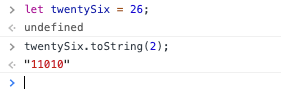toString() method to turn a decimal number into a binary number

We can see that the binary representation of the number 26 is “11010”, which is also the answer we got above when we converted the number by hand.

## parseInt()

Now, let’s see how we can transform numbers using the parseInt() method! This is more often used to convert numbers of different bases to Number primitives. The syntax for the parseInt() method is:

parseInt(Number, base of Number currently);

Since we transformed a decimal into a binary number using the toString() method, let’s use the parseInt() method to find the decimal value of a binary number. Below is an example of us converting “11010” to the decimal 26.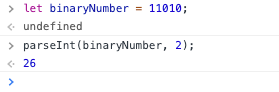Using parsInt() method to convert 11010 binary number to a decimal

We can see from the example, the number 11010 is established as binary in the second parameter (base 2), which gives binaryNumber a value of 26. converted to the decimal number 26 when it is given the base of 2 to translate the binary number.

Before we move on into bitwise operations, I want to make it clear that when using toString() and parseInt(), they are not interchangeable. We use toString() when we are trying to convert a base 10 number (decimal) to a Number of a different base. We use the parseInt() method when we are attempting to change numbers of different bases back into a decimal or Number with base 10. As long as you understand that, transforming Numbers is not too difficult!

# Bitwise Operations and How To Manipulate Bits

Now that we know what bits are and how to transform any integer to a binary number, let’s get into some different operations that will help us manipulate bits and have them work for us. I will be using the operations in examples and I encourage you to follow along!

## Slight But Important Detour

Before we get started with bitwise operations, there is an important note about binary numbers that I wanted to provide to you. I want to discuss how we write a negative number in binary. When establishing whether a binary number is positive or negative, we use what’s called a signed number. Signed binary numbers use the leftmost digit to determine whether the the number is positive or negative. We can also refer to the leftmost digit as the MSB or “most significant bit”. If the binary number is negative the MSB will be 1, and if it is positive the MSB will be 0. Now that you know how to represent negative and positive binary numbers let’s go through some different bitwise operations that will help you manipulate some bits!

## Bitwise Operations

First, it’s important to know that in JavaScript when bitwise operations are performed the numbers are converted from 64-bit numbers to 32-bit signed integers. So when I told you earlier that JavaScript represents numbers using 64-bits, once a bitwise operation is used to manipulate that number, it is converted into a 32-bit signed number. It’s important to keep this in mind in case the representations of numbers you use at first have a different number of bits after you use the bitwise operations. JavaScript does this to guarantee that binary numbers that you operate with will always have the same number of bits and help you identify positive and negative integers. Now, let’s start with the & (AND) operator!

## & (AND) Operator

The bitwise AND operator (&), when applied to 2 binary numbers, will return 1 whenever the corresponding bits of both are 1. Let’s look at our example below to get a better understanding of what’s happening!

Let’s start where I declare the constant twelve and twentySeven as binary numbers using the “0b” prefix before the binary representations of the numbers. Then I declare the value of and as twelve & twentySeven, using the & bitwise operator, and received a value of 8 (decimal). How did we get 8? Well, let’s take both binary numbers and break down what is actually happening.

twelve = 1100

twentySeven = 11011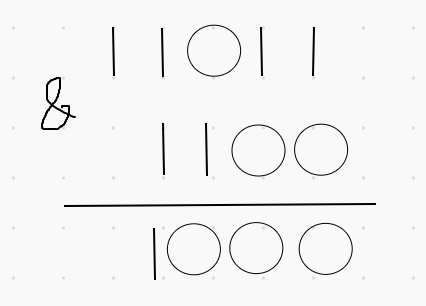How we got the binary number for 8 from twelve & twentySeven

If you see from the example above, the & operator takes both binary numbers and compares corresponding digits to return the binary number 1000. If the corresponding digits are both 1, the operator will return a 1, otherwise it will return a 0. The binary number 1000 represents the value of 8 in decimal terms and thus the value of and is 8. We can test this out by using the toString() method, which we learned how to use above, to return the binary number representation of the variable and.

## | (OR) Operator

The OR operator (|) is a bitwise operator that will return 1 whenever one of the corresponding bits of the operand is 1. Let’s use the same example as above, but use the OR operator to see how it works.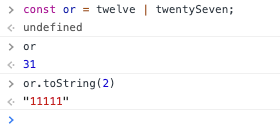OR bitwise Operator returning 31 or 11111.

So from the console above, when we use the OR operator with twelve and twentySeven, we get the decimal number of 31. Let’s got to the drawing board to see how we got that!

twelve = 1100

twentySeven = 11011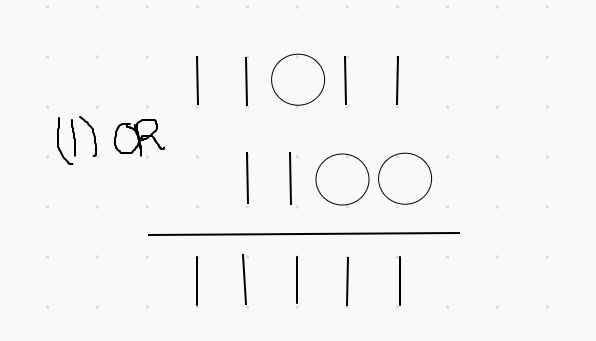Using the OR operator to return the answer of 31

We go back to our board and now it will return 1 whenever at least one of the digits contains a 1. You can see from the return result above that we get the binary number of 11111. If we convert 11111 into a decimal we get 31, which is why the variable or in the console above returns a value of 31. We can check that the math in our drawing board was correct by calling the toString() method on or and returning the binary representation of 31, which we can see is 11111.

## ^(XOR) Operator

The XOR operator, also known as the exclusive OR, is an operator that will return 1 whenever the two corresponding bits are different from each other. This means that any combination of zeros and ones will result in 1. Let’s take our example above and use the XOR operator on twelve and twentySeven.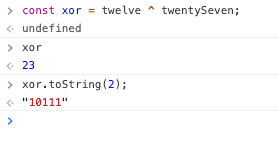XOR operator being used on twelve and twentySeven to get 23 or 10111

We can see from the example above that when we use the XOR operator above on twelve and twentySeven, we get a value of 23 or in binary 10111. Let’s break the return value down like we have for the last two operators on the drawing board!

twelve = 1100

twentySeven = 11011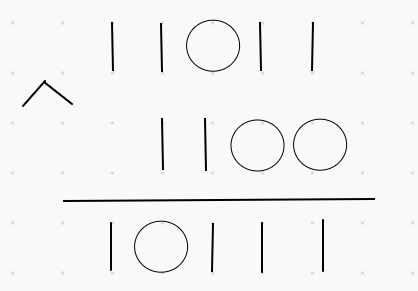Drawing board proof of how the XOR operator works on binary numbers

We can see from the drawing board that when comparing the corresponding digits, whenever the two numbers are different from each other a 1 is returned, and when they are the same a 0 is returned. This is how the value of the xor constant is equal to 23 because its binary representation is 10111. We can check that we completed the proof above correctly by calling the toString() method on xor.

## ~(NOT) Operator

The last operator I want to go over in this blog is the NOT operator. It is a pretty simple operator because all it does is invert the operand. This means when the digit is 1 it will return a 0 and vice versa. Let’s look at the NOT operator in action with our example.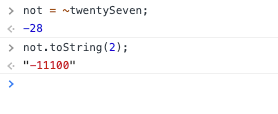Using the NOT Operator on twentySeven

When you look at our console, we establish that the not variable is equal to the inverse of twentySeven, which we see is -28. The NOT operator transforms positive numbers into negative numbers and vice versa because as I mentioned earlier, we use the leftmost bit of a binary number to indicate its signal. But, this does not mean that we simply add a 1 or zero before the number, the operator is applied to each bit of a number individually. So, of the binary number 11011 (27, decimal) the return value would be 00100. The return value of a NOT operator or the inverse of the Number is also known as its complement.

# Conclusion

In this week’s blog we’ve learned what bits are and why they are important. We also began reviewing some of the basic bitwise operations for bit manipulation in JavaScript. I hope this blog was informational and helpful for you as you continue learning about data structures and algorithms. Part 2 will come out next week and discuss some more advanced operations that will review shifting and adding and subtracting binary numbers. As always, I challenge you to continue researching and practicing bit manipulation and come back next week for part 2!

--

--